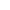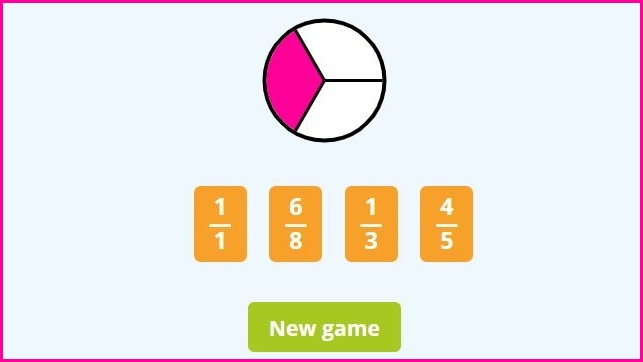﻿ Identifying Fractions Games + Worksheets

Page No. 1041

# Identifying fractions games

Play identifying fractions games online. matching fractions to pictures. identify fractions of shapes. Identifying fractions worksheets PDF. Matching fractions to pictures. identifying fractions game. recognising fractions. Identifying fractions using blocks. Learn to identify fractions. Your task is to choose the correct fraction by clicking on it. identifying fractions online games. Download fraction identification worksheets in PDF. identify fractions game. identifying fractions practice. identifying fractions pdf.

New gameWhat fraction of the shape is shaded?

## Identifying fractions games ONLINEA fraction is part of a whole number (or shape or set). Your task is to choose the correct fraction by clicking on it. Press the button "New game" and you can play a new identifying fractions game.

## Identifying fractions worksheets PDF

Identifying fractions games complement well with these identifying fractions worksheets in PDF. They are generated automatically, so you can download as many fraction identification worksheets as you want.

Click the image bellow and download the free identifying fractions worksheets in PDF:

These fraction identification worksheets contain various fractions in the range from 1 (1/1) to 10/10. You can find more printable fractions worksheets here.

## Identify fractions of shapes

The objective of the fraction game is to identify the correct fraction that corresponds to the shaded portion of a given shape. If you're only beginning to identify fractions, then consider watching this YouTube video or this YouTube video on that topic.

### How to identify fractions of shapes

Here are some instructions how to play ´Identify fractions of shapes´game:

1. A picture of a shape will be shown on the screen, with a certain portion of the shape shaded.
2. Four fraction options will be given (e.g. 1/2, 1/3, 1/4, 1/5), one of which is the correct answer to the question "What fraction of the shape is shaded?"
3. You must choose the correct fraction by clicking on it. If you select the incorrect answer, you have to try again.
4. The game continues with different shapes and fractions as long as you enjoy the game.
5. Here is an example: A picture of a rectangle is shown on the screen, with 2/3 of the rectangle shaded in. The four fraction options given are 1/2, 2/3, 3/4, and 4/5. The correct answer would be 2/3, so the player would click on that option to move on to the next question.

## Matching fractions to pictures

Identifying fractions of shapes involves determining what fraction of a geometric shape is shaded or colored in. For example, if a rectangle is divided into four equal parts and one part is shaded, then the fraction of the rectangle that is shaded is 1/4.### Identifying fractions game

I created online identifying fractions game to provide fun and easy practice of fraction identification. Kids love matching fractions to pictures: to circles or fraction bars.

Remember that the fraction represents the shaded portion of the shape compared to the whole shape. The best way to introduce fractions to beginners is to use these fraction flashcards.

## Identifying fractions using blocks

Fraction identification is a crucial skill that children must have in order to understand identifying fractions on a number line.

## Identify the fraction game

Did you know that you come across fractions in your daily life without even realizing it? In this ´Identify the fraction game´ you will learn how to identify fractions.

Let´s take an example you are familiar with: a pizza. Pizza is usually cut into equal parts. These are fractions. Fraction is part of whole (or shape or whole set of things: for example bag of jellybeans).

### How to identify fractions?

If the pizza is cut into 4 slices and you eat 3 slices, you have eaten 3/4 of the pizza. 3/4 is a fraction.

Fractions are written in a special way. The bottom number (called denominator) tells how many parts the whole is divided, in our case the pizza is cut into 4 parts. The top number (called numerator) tells how many pieces we are talking about (eaten). So we say that three fourths of the pizza was eaten.

### Identifying fractions online games

In our identifying fractions game we ask: What fraction of the shape (circle or rectangle) is shaded?

First you count how many parts the shape has: this number will be in the bottom part of the fraction. Then you count how many parts are colored: this number will be at the top of the fraction.

Category: